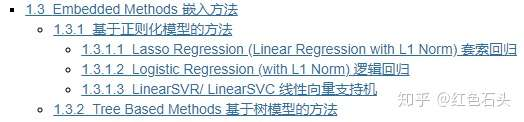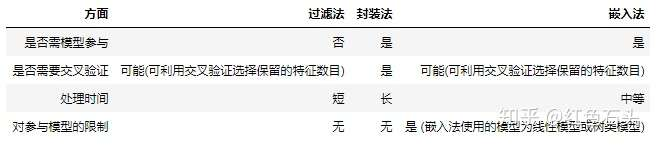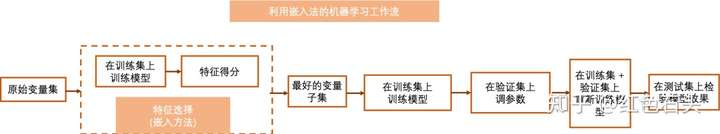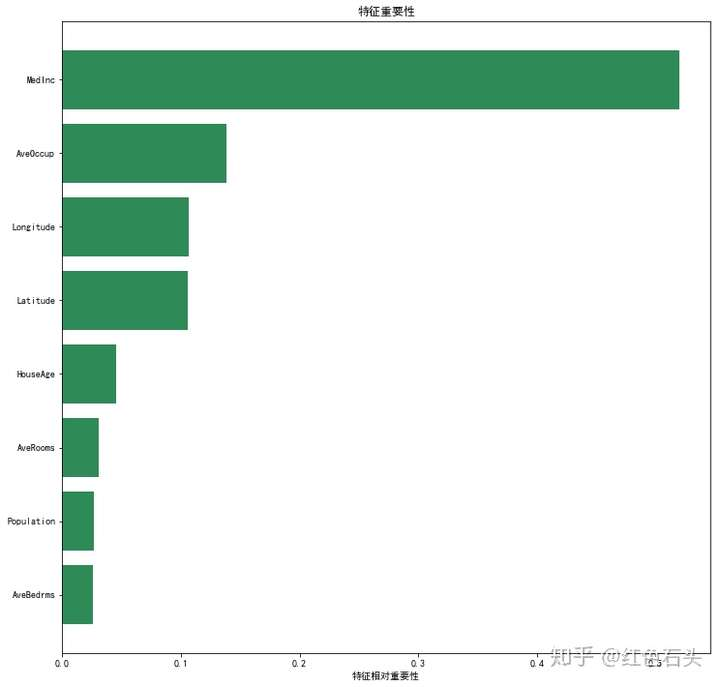# 专栏 | 基于 Jupyter 的特征工程手册：特征选择（五）### 1.3.1 基于正则化模型的方法

#### 1.3.1.1 Lasso Regression (Linear Regression with L1 Norm) 套索回归

import numpy as np
from sklearn.feature_selection import SelectFromModel
from sklearn.linear_model import Lasso # 我们也可以使用带L2正则项的岭回归

# 直接载入数据集
from sklearn.datasets import fetch_california_housing
dataset = fetch_california_housing()
X, y = dataset.data, dataset.target # 利用 california_housing 数据集来演示

# 选择前15000个观测点作为训练集
# 剩下的作为测试集
train_set = X[0:15000,:]
test_set = X[15000:,]
train_y = y[0:15000]

clf = Lasso(normalize=True, alpha = 0.001)
# 在进行线性回归前，我们需要先对变量进行缩放操作，否则回归系数大小无法比较
# alpha控制正则效果的大小，alpha越大，正则效果越强

clf.fit(train_set, train_y) # 在训练集上训练
np.round(clf.coef_ ,3)


array([ 0.346, 0.003, -0. , -0. , -0. , -0. , -0.033, 0. ])

selector = SelectFromModel(clf, prefit=True, threshold=1e-5)
# 阈值被设置为1e-5，因此绝对系数低于1e-5的特征将被删除
# 我们还可以设置max_features参数以选择最重要的前几个特征

transformed_train = selector.transform(train_set) # 转换训练集
transformed_test = selector.transform(test_set) #转换测试集

assert np.array_equal(transformed_train, train_set[:,[0,1,6]])
# 选择第一个，第二个及第七个变量
assert np.array_equal(transformed_test, test_set[:,[0,1,6]])


#### 1.3.1.2 Logistic Regression (with L1 Norm) 逻辑回归

import numpy as np
from sklearn.feature_selection import SelectFromModel
from sklearn.linear_model import LogisticRegression
from sklearn.datasets import load_iris  # 利用iris数据作为演示数据集

# 载入数据集
X, y = iris.data, iris.target

# iris 数据集使用前需要被打乱顺序
np.random.seed(1234)
idx = np.random.permutation(len(X))
X = X[idx]
y = y[idx]

# 选择前100个观测点作为训练集
# 剩下的50个观测点作为测试集
train_set = X[0:100,:]
test_set = X[100:,]
train_y = y[0:100]

# 在进行逻辑回归前，我们需要先对变量进行缩放操作，否则回归系数大小无法比较
from sklearn.preprocessing import StandardScaler
model = StandardScaler()
model.fit(train_set)
standardized_train = model.transform(train_set)
standardized_test = model.transform(test_set)

clf = LogisticRegression(penalty='l1', C = 0.7,
random_state=1234, solver='liblinear')
# 我们也可以将正则项设置为 'l2'
# C控制正则效果的大小，C越大，正则效果越弱

clf.fit(standardized_train, train_y)
np.round(clf.coef_,3)


array([[ 0. , 1. , -3.452, -0.159],
[ 0. , -1.201, 0.053, 0. ],
[ 0. , 0. , 1.331, 3.27 ]])

selector = SelectFromModel(clf, prefit=True, threshold=1e-5)
# 阈值被设置为1e-5，因此绝对系数低于1e-5的特征将被删除
# 我们还可以设置max_features参数以选择最重要的前几个特征

transformed_train = selector.transform(train_set) # 转换训练集
transformed_test = selector.transform(test_set) #转换测试集

assert np.array_equal(transformed_train, train_set[:,[1,2,3]])
# 选择第2个, 第3个及第4个变量
assert np.array_equal(transformed_test, test_set[:,[1,2,3]])


#### 1.3.1.3 LinearSVR/ LinearSVC 线性向量支持机

# LinearSVC 用于分类问题
# LinearSVR 用于回归问题
# 这里以LinearSVR为例

import numpy as np
from sklearn.feature_selection import SelectFromModel
from sklearn.svm import LinearSVR

# 直接载入数据集
from sklearn.datasets import fetch_california_housing
dataset = fetch_california_housing()
X, y = dataset.data, dataset.target # 利用 california_housing 数据集来演示

# 选择前15000个观测点作为训练集
# 剩下的作为测试集
train_set = X[0:15000,:]
test_set = X[15000:,]
train_y = y[0:15000]

# 在进行逻辑回归前，我们需要先对变量进行缩放操作，否则回归系数大小无法比较
from sklearn.preprocessing import StandardScaler
model = StandardScaler()
model.fit(train_set)
standardized_train = model.transform(train_set)
standardized_test = model.transform(test_set)

clf = LinearSVR(C = 0.0001, random_state = 123)
# C控制正则效果的大小，C越大，正则效果越弱

clf.fit(standardized_train, train_y)
np.round(clf.coef_,3)


array([ 0.254, 0.026, 0.026, -0.017, 0.032, -0.01 , -0.1 , -0.037])

selector = SelectFromModel(clf, prefit=True, threshold=1e-2)
# 阈值被设置为1e-2，因此绝对系数低于1e-2的特征将被删除
# 我们还可以设置max_features参数以选择最重要的前几个特征

transformed_train = selector.transform(train_set) # 转换训练集
transformed_test = selector.transform(test_set) #转换测试集

assert np.array_equal(transformed_train, train_set[:,[0,1,2,3,4,6,7]])
# 仅第6个变量被删去
assert np.array_equal(transformed_test, test_set[:,[0,1,2,3,4,6,7]])


### 1.3.2 Tree Based Methods 基于树模型的方法

https://github.com/YC-Coder-Chen/Tree-Math

# 我们以随机森林为例
import numpy as np
from sklearn.feature_selection import SelectFromModel
from sklearn.ensemble import RandomForestRegressor

# 直接载入数据集
from sklearn.datasets import fetch_california_housing
dataset = fetch_california_housing()
X, y = dataset.data, dataset.target # 利用 california_housing 数据集来演示

# 选择前15000个观测点作为训练集
# 剩下的作为测试集
train_set = X[0:15000,:]
test_set = X[15000:,]
train_y = y[0:15000]

# 在树类机器学习模型中，我们无需缩放变量操作

clf = RandomForestRegressor(n_estimators = 50, random_state = 123)

clf.fit(train_set, train_y)
np.round(clf.feature_importances_, 3)


array([0.52 , 0.045, 0.031, 0.026, 0.027, 0.139, 0.106, 0.107])

# 可视化特征重要性
import matplotlib.pyplot as plt
plt.rcParams['font.sans-serif']=['SimHei']
%matplotlib inline
importances = clf.feature_importances_
indices = np.argsort(importances)
plt.figure(figsize=(12,12))
plt.title('特征重要性')
plt.barh(range(len(indices)), importances[indices], color='seagreen', align='center')
plt.yticks(range(len(indices)),np.array(dataset.feature_names)[indices])
plt.xlabel('特征相对重要性');selector = SelectFromModel(clf, prefit=True, threshold='median')
# 阈值被设定为'median', 即以特征重要性的中位数作为阈值，大约为0.076
# 我们还可以设置max_features参数以选择最重要的前几个特征

transformed_train = selector.transform(train_set)
transformed_test = selector.transform(test_set)
assert np.array_equal(transformed_train, train_set[:,[0,5,6,7]])
# 选择来第1个，第6个, 第7个及第8个特征
assert np.array_equal(transformed_test, test_set[:,[0,5,6,7]])### 觉得文章有用就打赏一下文章作者

#### 支付宝扫一扫打赏#### 微信扫一扫打赏